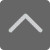# 小学口算题大全：二年级100以内加减法口算题（四）

2018-11-02 16:33:39 　来源：网络 文章作者：网络
 免费下载 3个G小报手抄报电子版 小学1-5年级上册期末复习资料 [ 戳我免费下载 ]

94-56=

12+9+41=

44-18=

63-17=

4+22+8=

15+63=

42-27=

33+2+38=

49+39=

71+16=

77+21+9=

97-83=

71-59=

82+7+3=

76-65=

63-3=

62+5+15=

51+15=

54+12=

80+8+2=

73-52=

69+17=

59+30+10=

53+17=

63-7=加入QQ群，与更多家长交流经验！！！
 上海小学交流群：639215153 上海初中交流群：551802726 上海高中交流群：591337342 上海幼升小交流群：772707735